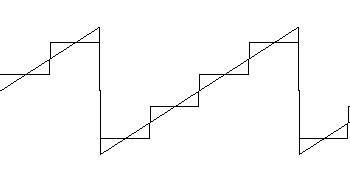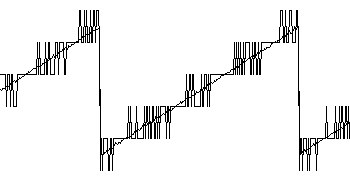Daqarta
Data AcQuisition And Real-Time Analysis
Scope - Spectrum - Spectrogram - Signal Generator
Software for WindowsThe following is from the Daqarta Help system:

# Dither: Noise + Averaging = Resolution

Although we are seldom happy about the need for averaging to reduce the noise in a signal, we can actually gain a hidden benefit from this situation in the form of increased bits of resolution. Although it may sound incredible at first, the addition of noise to an otherwise clean signal is sometimes done deliberately to take advantage of this phenomenon, known as "dither". Here's the scoop:

Most real-world signals are analog, and change smoothly between different voltages, whereas an ADC output is digital and can only have discrete values. To approximate the analog waveform, the ADC uses lots of very tiny steps. The maximum number of steps, and hence the size of the smallest step, is determined by the number of bits of the ADC. The typical 16-bit ADC in most sound cards has 2^16 or 65536 steps to cover any given input voltage range.

This is ample for large signals, where the steps are small compared to the signal itself. But when the signal is small, the steps are a greater percentage of the total range and the approximation is not so good. Consider a small ramp signal that only covers a few ADC steps; the smooth ramp input is converted to a chunky staircase:Since the ADC output can only take on the discrete steps of the minimum step size of the ADC, all information about intermediate values is lost. If you synchronously average a number of repetitions of the noise-free waveform, you get exactly the same chunky staircase. (Note that this has nothing to do with sample rate, only the ADC resolution: Sampling faster would just mean more samples at each step.)

But now suppose we add random noise to the input signal. If the peak-to-peak level of the noise is equal to the minimum step size, we find that each step of the staircase has "grass" growing out of it, where the noise plus the signal exceeds the ADC's threshold for the next step. Since the noise is random, the grass is different on each step, and on each repetition of the waveform. If we synchronously average many repetitions of the signal, the values between steps will be proportional to the average percent of grass:This shows a snapshot of the sampled waveform with the grass on each step. The diagonal lines may look like the original ramp, but they are actually the results of averaging 256 repetitions of the grassy combined waveform, where the grass was different on each repetition because it came from a random source.

Notice that the noise causes frequent transitions in regions that were the edges of the original stairs in the prior image, and fewer transitions near the center of each stair. It turns out that the center of each original stair is the desired true value of the ramp, which is unchanged by averaging since there are no noise-induced spikes at that point on any repetition. The noisy transition regions, on the other hand, average out to values midway between adjacent steps, forming a near-prefect replica of the original input ramp.

To see what's going on in detail, consider that the ADC has transition points midway between each output step. For purposes of this discussion, let's assume the ADC resolution is only 10 mV. (It's typically about 100 times better than that.) If the input is above 5 mV but less than 15 mV, the output will have only the least-significan bit (LSB) set, indicating a 10 mV level for anything in this entire range. When the input moves above 15 mV, the ADC output reports 20 mV. Hence, without dither, we get the staircase of the original image, with steps of 10 mV each.

Now consider the addition of a dither signal whose maximum excursion is +/-0.5 LSB, or +/-5 mV. Where the input ramp is exactly at 10 mV, the added noise will very rarely be at its extreme limits to bump the total above the 15 mV or below the 5 mV thresholds. Thus we see no "grass" at the center points of each bit value.

On the other hand, where the input ramp is just at the 5 mV transition point, any added noise will force the output either up to the 10 mV step or down to the 0 mV step, so we see a lot of output transitions here. If the noise has an average value of 0, we expect about half of the output values to be 10 mV and half 0, so after a lot of repetitions we expect the average output value to be pretty close to 5 mV ... even though the ADC itself can't resolve this value directly.

Similarly, intermediate values of the input ramp will produce intermediate averaged values. For example, if the ramp is at 6 mV, the ramp-plus-noise will run from 1 mV to 11 mV. Assuming a uniform distribution of noise values, we would expect about 40% of the noise values to be lower than -1 mV, such that the total input would be below 5 mV and thus the output would be 0. About 60% should be higher, giving an output of 10 mV. The average value then comes out to 6 mV, just as desired.

The improvement in resolution is proportional to the number of repetitions that are averaged together. If you average only 2 observations that are each either 0 or 10 mV, the only possible average values are 0, 5, or 10 mV. If you average 4 observations, you can get 0, 2.5, 5, 7.5, or 10 mV. Essentially, each doubling of the number of repetitions adds one bit of resolution by cutting the effective output step size in half.

There is no particular limit to this ability to increase resolution, except the practicality of how long you want to wait for the next bit. Adding an additional 8 bits of resolution only takes 256 repetitions; adding 16 bits takes 65536.

However, there is still the issue of the dither noise itself, which typically subsides as the square root of the number of repetitions averaged. Doubling the repetitions will double the resolution, but it will only cut the noise by 3 dB. Thus, the residual noise will be the limiting factor on true resolution, which improves much more slowly; you'll quickly get high apparent resolution, but the fine detail you see will just be noise until you really build up the repetitions. While the dither phenomenon may be a case where Nature finally gets generous, She's not that generous.

The previous discussion also assumes noise with an amplitude of exactly +/- 0.5 LSB and a uniform distribution. If the noise level is higher, the dither process still works, but there will be a higher noise level in the averaged output. Since many real-world signals are contaminated with enough noise to require averaging simply for noise reduction, the side effect of improved resolution may be considered a pure bonus.

See the Dither Demonstration topic for an easy-to-use hands-on example of dither in an audio signal, with screen shots.GO: